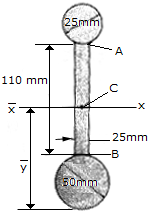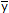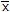# Engineering Mechanics - Center of Gravity and Centroid - Discussion

### Discussion :: Center of Gravity and Centroid - General Questions (Q.No.2)

2.Determine the distanceto the centroid axisof the beam's cross-sectional area. Neglect the size of the corner welds at A and B for the calculation.

 [A].= 75.2 mm [B].= 97.5 mm [C].= 85.9 mm [D].= 102.5 mm

Explanation:

No answer description available for this question.

 Khalid Anwar said: (Dec 23, 2013) What is the use of centroid?

 Dasari Krishnarao said: (Dec 27, 2013) What is the centroid for human body?

 Addisu said: (Feb 8, 2014) The use of centroid is. It tells you at what point the given object is in equilibrium (balance).

 Jaylord Proxy said: (Mar 7, 2014) I need to find the centroid of the human body for both a standing and seated position as part of design that I am putting together for an adaptive wheelchair design for a handicapped individual. If anybody can please explain to me how to go about finding the overall centroid of a seated and standing human being I would greatly appreciate it. FROM Michael~S: I am assuming that you just make an assumption that the limbs and torso can be approximated as rectangular sections, with the head approximated as a circle. If someone can give me a quick calculation or run-through of how this would be done, I would greatly appreciate it. Thanks for your assistance.

 Dharmalingam Ramasamy said: (Jul 11, 2016) a1 = pixr^2 = pix25^2 = 1963.5; a2 = 110 * 25 = 2750; a3 = pixr^2 = pix12.5^2 = 491; y1 = 25; y2 = 105; y3 = 172.5. = (a1y1 + a2y2 + a3y3) / (a1 + a2 + a3) = 85mm.

 Jaspreet Singh Nagpal said: (Sep 22, 2016) My Answer does not match with the given options. The correct answer is 81.19 please do check the calculations once again.

 Abhishek Sharma said: (Sep 27, 2016) The Correct answer is 81.18647324...

 Prashant said: (Oct 3, 2016) I think 81.2 mm is the correct answer.

 Mithun Raju N said: (Jan 9, 2017) However the nearest available option is 85.9 for 81.18. So we need select the option C.

 Ajit Kumar said: (Feb 3, 2018) 81.20 is the correct answer.

 Thompson Victor said: (Apr 10, 2018) Please tell, how did you get y3?

 Shashi said: (Nov 13, 2018) @Thompson Victor. y3 is measured from base to half of the top circle. i.e dia 25 mm so half means 12.5mm therefore from base 172.5= 50+110+12.5.